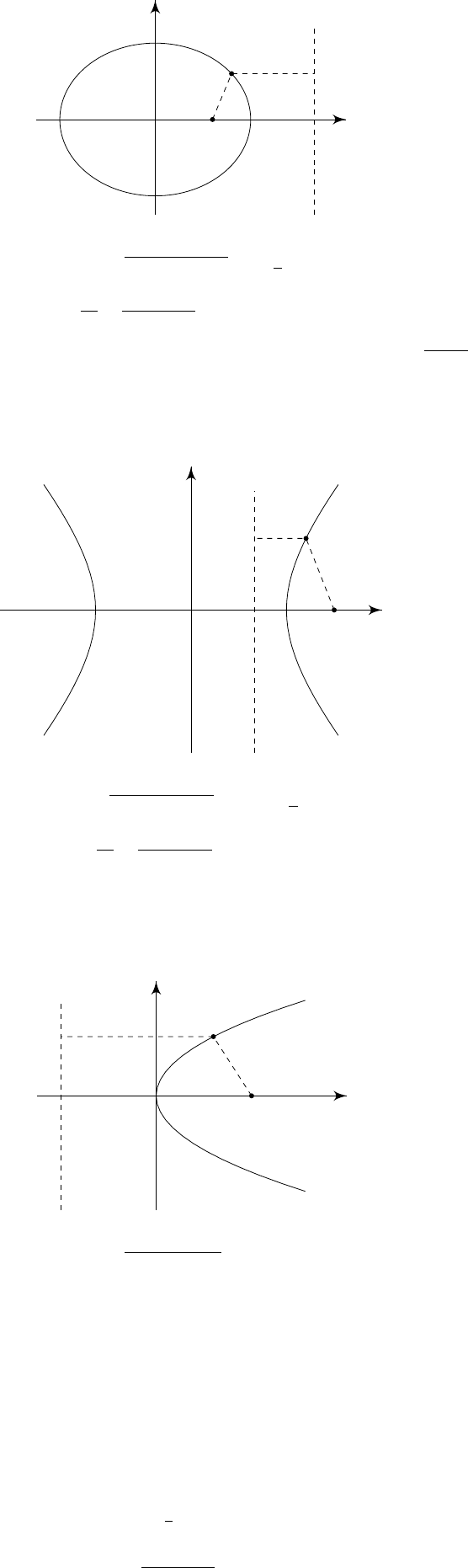IA Vectors and Matrices6.2 Focus-directrix property
Conic sections can be defined in a different way, in terms of
Definition
(Conic sections)
.
The eccentricity and scale are properties of a conic
section that satisfy the following:
Let the foci of a conic section be (±ae, 0) and the directrices be x = ±a/e.
A conic section is the set of points whose distance from focus is
e×
distance
from directrix which is closer to that of focus (unless
e
= 1, where we take the
distance to the other directrix).
Now consider the different cases of e:
(i) e < 1. By definition,
x
y
O
x = a/e
ae
(x, y)
p
(x ae)
2
+ y
2
= e
a
e
x
x
2
a
2
+
y
2
a
2
(1 e
2
)
= 1
Which is an ellipse with semi-major axis
a
and semi-minor axis
a
1 e
2
.
(if e = 0, then we have a circle)
(ii) e > 1. So
x
y
O
x = a/e
ae
(x, y)
p
(x ae)
2
+ y
2
= e
x
a
e
x
2
a
2
y
2
a
2
(e
2
1)
= 1
and we have a hyperbola.
(iii) e = 1: Then
x
y
O
x = a
a
(x, y)
p
(x a)
2
+ y
2
= (x + 1)
y
2
= 4ax
and we have a parabola.
Conics also work in polar coordinates. We introduce a new parameter
l
such
that l/e is the distance from the focus to the directrix. So
l = a|1 e
2
|.
We use polar coordinates (
r, θ
) centered on a focus. So the focus-directrix
property is
r = e
l
e
r cos θ
r =
l
1 + e cos θ
We see that
r
if
θ cos
1
(
1
/e
), which is only possible if
e
1, i.e.
hyperbola or parabola. But ellipses have e < 1. So r is bounded, as expected.